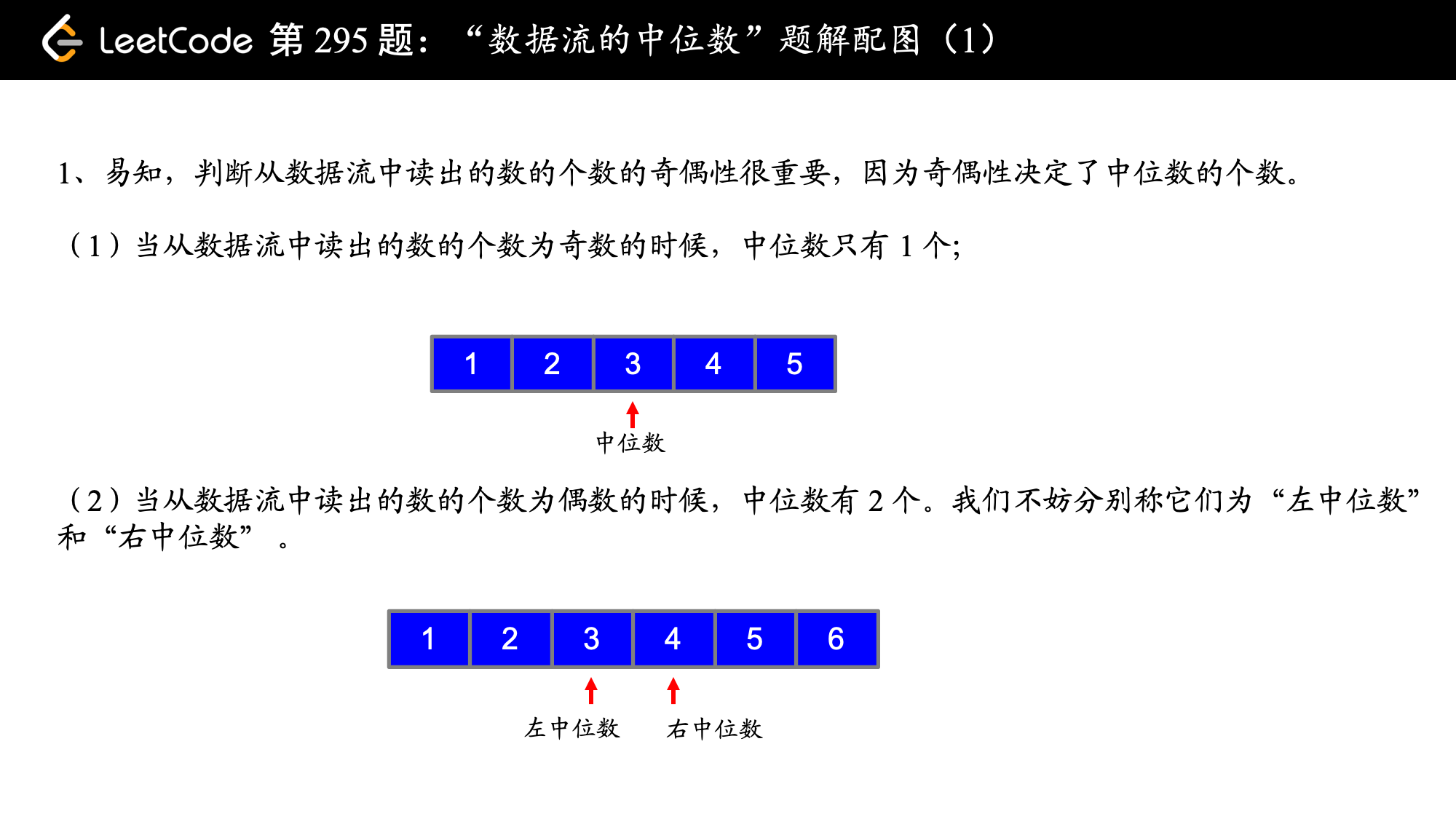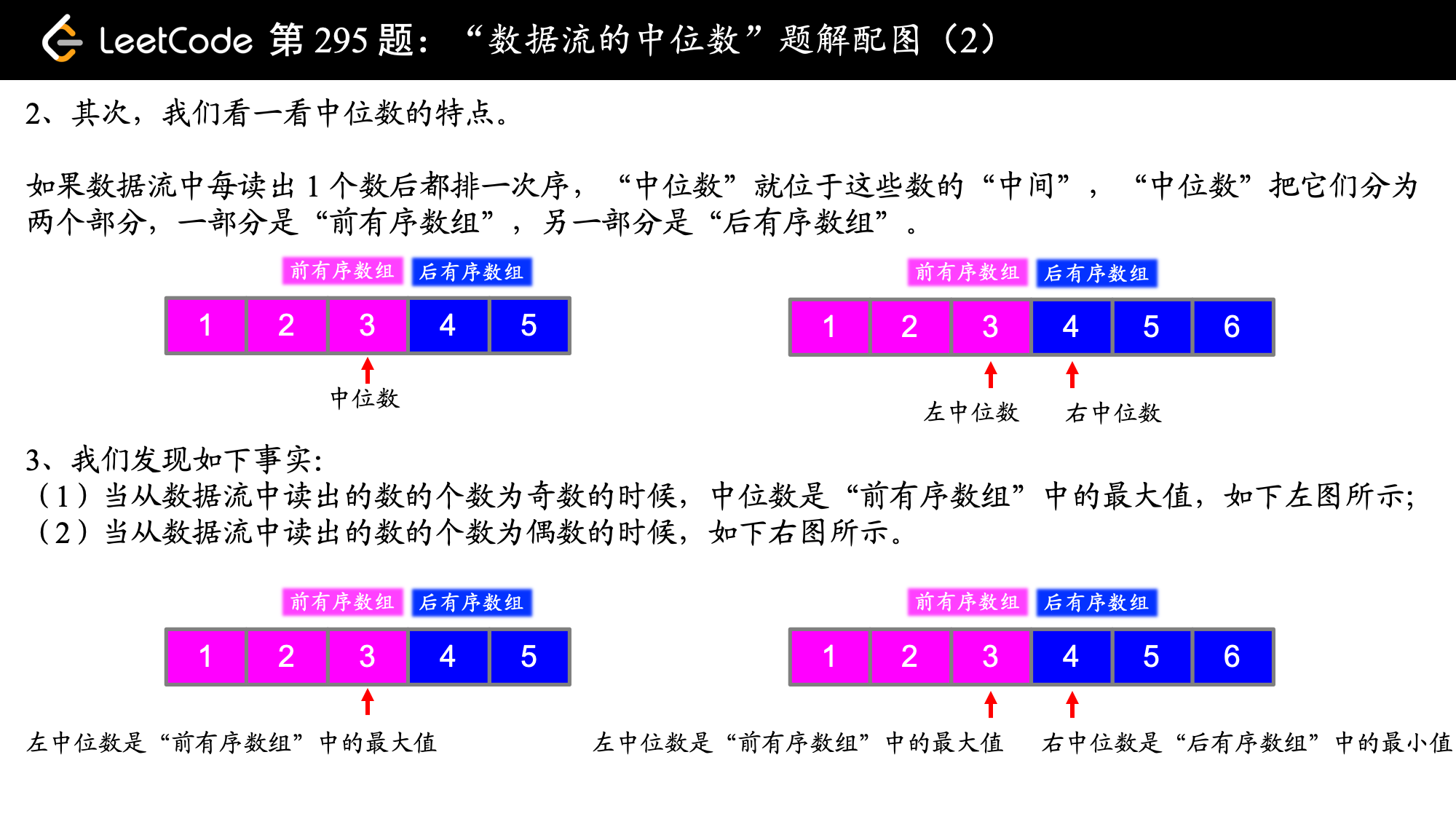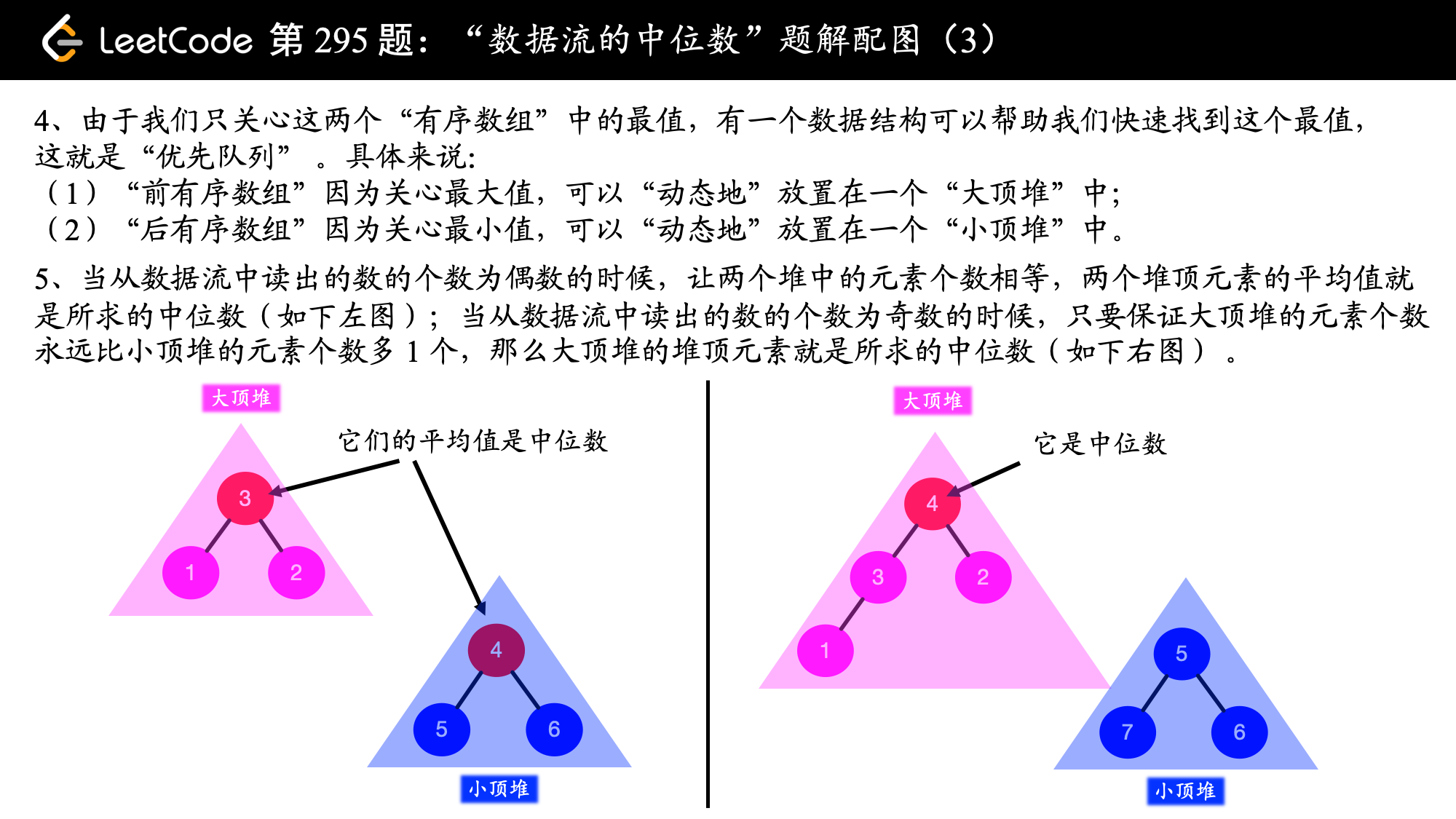# 「力扣」第 295 题：数据流的中位数（中等）

## 「力扣」第 295 题：数据流的中位数（中等）

[2, 3, 4] 的中位数是 3

[2, 3] 的中位数是 (2 + 3) / 2 = 2.5

• void addNum(int num) - 从数据流中添加一个整数到数据结构中。
• double findMedian() - 返回目前所有元素的中位数。
示例：
addNum(1)
findMedian() -> 1.5
findMedian() -> 2

1. 如果数据流中所有整数都在 $0$ 到 $100$ 范围内，你将如何优化你的算法？
2. 如果数据流中 $99%$ 的整数都在 $0$ 到 $100$ 范围内，你将如何优化你的算法？),),),1、数据是如何在两个堆之间流动的，脑子里要建立如下动态的过程：为了找到添加新数据以后，数据流的中位数，我们让这个新数据在大顶堆和小顶堆中都走了一遍。而为了让大顶堆的元素多 $1$ 个，我们让从小顶堆中又拿出一个元素“送回”给大顶堆

2、将元素放入优先队列以后，优先队列会以对数时间复杂度自行调整，把“最优值”放入堆顶，这是使用优先队列解决这个问题的原因。如果不太熟悉优先队列的朋友们，请复习一下优先队列的相关知识，包括基本操作，理解上浮和下沉。

Java 代码：

import java.util.PriorityQueue;

public class MedianFinder {

/**
* 当前大顶堆和小顶堆的元素个数之和
*/
private int count;
private PriorityQueue maxheap;
private PriorityQueue minheap;

/**
* initialize your data structure here.
*/
public MedianFinder() {
count = 0;
maxheap = new PriorityQueue<>((x, y) -> y - x);
minheap = new PriorityQueue<>();
}

count += 1;
maxheap.offer(num);
// 如果两个堆合起来的元素个数是奇数，小顶堆要拿出堆顶元素给大顶堆
if ((count & 1) != 0) {
}
}

public double findMedian() {
if ((count & 1) == 0) {
// 如果两个堆合起来的元素个数是偶数，数据流的中位数就是各自堆顶元素的平均值
return (double) (maxheap.peek() + minheap.peek()) / 2;
} else {
// 如果两个堆合起来的元素个数是奇数，数据流的中位数大顶堆的堆顶元素
return (double) maxheap.peek();
}
}
}

Python 代码：

import heapq

class MedianFinder:

def __init__(self):
# 当前大顶堆和小顶堆的元素个数之和
self.count = 0
self.max_heap = []
self.min_heap = []

def addNum(self, num: int) -> None:
self.count += 1
# 因为 Python 中的堆默认是小顶堆，所以要传入一个 tuple，用于比较的元素需是相反数，
# 才能模拟出大顶堆的效果
heapq.heappush(self.max_heap, (-num, num))
_, max_heap_top = heapq.heappop(self.max_heap)
heapq.heappush(self.min_heap, max_heap_top)
if self.count & 1:
min_heap_top = heapq.heappop(self.min_heap)
heapq.heappush(self.max_heap, (-min_heap_top, min_heap_top))

def findMedian(self) -> float:
if self.count & 1:
# 如果两个堆合起来的元素个数是奇数，数据流的中位数大顶堆的堆顶元素
return self.max_heap
else:
# 如果两个堆合起来的元素个数是偶数，数据流的中位数就是各自堆顶元素的平均值
return (self.min_heap + self.max_heap) / 2

# Your MedianFinder object will be instantiated and called as such:
# obj = MedianFinder()
# param_2 = obj.findMedian()

• 时间复杂度：$O(\log N)$，优先队列的出队入队操作都是对数级别的，数据在两个堆中间来回操作是常数级别的，综上时间复杂度是 $O(\log N)$ 级别的。

• 空间复杂度：$O(N)$，使用了三个辅助空间，其中两个堆的空间复杂度是 $O(\cfrac{N}{2})$，一个表示数据流元素个数的计数器 count，占用空间 $O(1)$，综上空间复杂度为 $O(N)$。

### 使用自己实现的“大顶堆” 和 “小顶堆”

Python 代码：

class MaxHeap:
def __init__(self, capacity):
# 我们这个版本的实现中，0 号索引是不存数据的，这一点一定要注意
# 因为数组从索引 1 开始存放数值
# 所以开辟 capacity + 1 这么多大小的空间
self.data = [None for _ in range(capacity + 1)]
# 当前堆中存储的元素的个数
self.count = 0
# 堆中能够存储的元素的最大数量（为简化问题，不考虑动态扩展）
self.capacity = capacity

def size(self):
"""
返回最大堆中的元素的个数
:return:
"""
return self.count

def is_empty(self):
"""
返回最大堆中的元素是否为空
:return:
"""
return self.count == 0

def insert(self, item):
if self.count + 1 > self.capacity:
raise Exception('堆的容量不够了')
self.count += 1
self.data[self.count] = item
# 考虑将它上移
self.__swim(self.count)

def __shift_up(self, k):
# 有索引就要考虑索引越界的情况，已经在索引 1 的位置，就没有必要上移了
while k > 1 and self.data[k // 2] < self.data[k]:
self.data[k // 2], self.data[k] = self.data[k], self.data[k // 2]
k //= 2

def __swim(self, k):
# 上浮，与父结点进行比较
temp = self.data[k]
# 有索引就要考虑索引越界的情况，已经在索引 1 的位置，就没有必要上移了
while k > 1 and self.data[k // 2] < temp:
self.data[k] = self.data[k // 2]
k //= 2
self.data[k] = temp

def extract_max(self):
if self.count == 0:
raise Exception('堆里没有可以取出的元素')
ret = self.data
self.data, self.data[self.count] = self.data[self.count], self.data
self.count -= 1
self.__sink(1)
return ret

def __shift_down(self, k):
# 只要有左右孩子，左右孩子只要比自己大，就交换
while 2 * k <= self.count:
# 如果这个元素有左边的孩子
j = 2 * k
# 如果有右边的孩子，大于左边的孩子，就好像左边的孩子不存在一样
if j + 1 <= self.count and self.data[j + 1] > self.data[j]:
j = j + 1
if self.data[k] >= self.data[j]:
break
self.data[k], self.data[j] = self.data[j], self.data[k]
k = j

def __sink(self, k):
# 下沉
temp = self.data[k]
# 只要它有孩子，注意，这里的等于号是十分关键的
while 2 * k <= self.count:
j = 2 * k
# 如果它有右边的孩子，并且右边的孩子大于左边的孩子
if j + 1 <= self.count and self.data[j + 1] > self.data[j]:
# 右边的孩子胜出，此时可以认为没有左孩子
j += 1
# 如果当前的元素的值，比右边的孩子节点要大，则逐渐下落的过程到此结束
if temp >= self.data[j]:
break
# 否则，交换位置，继续循环
self.data[k] = self.data[j]
k = j
self.data[k] = temp

class MinHeap:

# 把最大堆实现中不等号的方向反向就可以了

def __init__(self, capacity):
# 因为数组从索引 1 开始存放数值
# 所以开辟 capacity + 1 这么多大小的空间
self.data = [0 for _ in range(capacity + 1)]
self.count = 0
self.capacity = capacity

def size(self):
return self.count

def is_empty(self):
return self.count == 0

def insert(self, item):
if self.count + 1 > self.capacity:
raise Exception('堆的容量不够了')
self.count += 1
self.data[self.count] = item
self.__swim(self.count)

def __swim(self, k):
# 上浮，与父节点进行比较
temp = self.data[k]
while k > 1 and self.data[k // 2] > temp:
self.data[k] = self.data[k // 2]
k //= 2
self.data[k] = temp

def extract_min(self):
if self.count == 0:
raise Exception('堆里没有可以取出的元素')
ret = self.data
self.data = self.data[self.count]
self.count -= 1
self.__sink(1)
return ret

def __sink(self, k):
# 下沉
temp = self.data[k]
while 2 * k <= self.count:
j = 2 * k
if j + 1 <= self.count and self.data[j + 1] < self.data[j]:
j += 1
if temp <= self.data[j]:
break
self.data[k] = self.data[j]
k = j
self.data[k] = temp

class MedianFinder:

def __init__(self):
"""
"""
# 如果测试用例的容量增加，下面 10000 这个数值请大家自行调整
self.max_heap = MaxHeap(10000)
self.min_heap = MinHeap(10000)

def addNum(self, num: 'int') -> 'None':
# 大顶堆先进一个元素
self.max_heap.insert(num);
# 然后从大顶堆里出一个元素到小顶堆
self.min_heap.insert(self.max_heap.extract_max())
if self.max_heap.size() < self.min_heap.size():
# 如果大顶堆的元素少于小顶堆
# 就要从小顶堆出一个元素到大顶堆
self.max_heap.insert(self.min_heap.extract_min())

def findMedian(self) -> 'float':
if self.max_heap.size() == self.min_heap.size():
return (self.max_heap.data + self.min_heap.data) / 2
else:
return self.max_heap.data

# Your MedianFinder object will be instantiated and called as such:
# obj = MedianFinder()
# param_2 = obj.findMedian()

上一篇「力扣」第 199 题：二叉树的右视图（中等）
「力扣」第 199 题：二叉树的右视图（中等）传送门：199. 二叉树的右视图。 给定一棵二叉树，想象自己站在它的右侧，按照从顶部到底部的顺序，返回从右侧所能看到的节点值。 示例: 输入: [1,2,3,null,5,null,4] 输出
2017-10-05「力扣」第 107 题：二叉树的层次遍历 II（中等）
「力扣」第 107 题：二叉树的层次遍历 II（中等）传送门：107. 二叉树的层次遍历 II。 给定一个二叉树，返回其节点值自底向上的层次遍历。 （即按从叶子节点所在层到根节点所在的层，逐层从左向右遍历） 例如：给定二叉树 [3,9,2
2017-10-04
目录